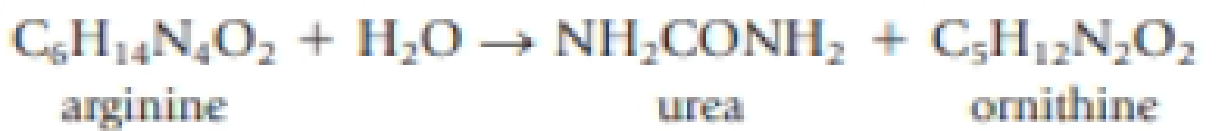Chapter 4, Problem 81GQ

Chapter
Section
Textbook Problem

Your body deals with excess nitrogen by excreting it in the form of urea, NH2CONH2. The reaction producing it is the combination of arginine (C6H14N4O2) with water to give urea and ornithine (C5H12N2O2).If you excrete 95 mg of urea, what mass of arginine must have been used? What mass of ornithine must have been produced?

Interpretation Introduction

Interpretation:

The mass of arginine used and the mass of ornithine produced when 95mg of urea excreted from the body has to be determined.

Concept introduction:

• The relation between the number of moles and mass of the substance is,

Number of mole = Mass in gramMolarmass

Mass of the substance=Numberofmole × Molarmass

• The molar mass of an element or compound is the mass in grams of 1 mole of that substance, and it is expressed in the unit of grams per mol (g/mol).
• For chemical reaction balanced chemical reaction equation written in accordance with the Law of conservation of mass.
• Law of conservation of mass states that for a reaction total mass of the reactant and product must be equal.
• Stoichiometric factor is a relationship between reactant and product which is obtained from the balanced chemical equation for a particular reaction.
Explanation

Balanced chemical equation for the given reaction is,

C6H14N4O2+H2ONH2CONH2+C5H12N2O2arginineurea ornithine

From the balanced chemical equation it is clear that arginine, urea and ornithine, react in a 1:1:1 ratio.

The amount (moles) of urea NH2CONH2  available in the reaction can be calculated as follows,

Amount of NH2CONH2 available =0.095gofNH2CONH260.06g/molNH2CONH2 = 0.0016molNH2CONH2

Using the stoichiometric factor the amount of arginine C6H14N4O2 can be calculated.

0

Still sussing out bartleby?

Check out a sample textbook solution.

See a sample solution

The Solution to Your Study Problems

Bartleby provides explanations to thousands of textbook problems written by our experts, many with advanced degrees!

Get Started

Find more solutions based on key concepts